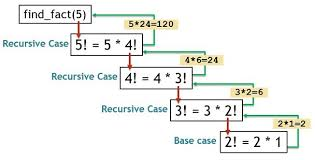Remove Left Recursion From The CFG In Python

Hello Friends, Today we are going to discuss on a Question which asked many times by My Followers…….

So now without any delay let’s start our topic on “Remove Left Recursion from the CFG in Python Programming Language”.

AlgorithmBefore we start our tutorial, we have to know the Algorithm,Let’s see it :-

N -->N / x / y

It is called left recursive where N is any non Terminal and x, and y are any set of terminals.

Problem with Left Recursion :-

If we use a left recursion in any of our grammar then, during parsing in the syntax analysis part of compilation there is a chance that the grammar will create infinite loop or you could say that it is craete a infinaite loop after the end of the program. This is because at every time of production of grammar N will produce another N without checking any condition.

Algorithm to Remove Left Recursion with example :-Assume we have a grammar which contains left recursion :-

N -->Nx / Ny / a / b

Check if the given grammar contains left recursion, if present then separate the production and start working on it.

In our example,

N -->Nx / Ny / a / b

Introduce a new nonterminal and write it at the last of every terminal. We produce a new nonterminal N’and write new production as,

Nn → aN' / bN'

Write newly produced nonterminal in LHS and in RHS it can either produce or it can produce new production in which the terminals or non terminals which followed the previous LHS will be replaced by new nonterminal at last.

N'-->? / xN' / yN'

So after conversion the new equivalent production is

N-->aN' / bN'
N'-->? / xN' / yN'

Indirect Left Recursion:-A grammar is said to have indirect left recursion if, starting from any symbol of the grammar, it is possible to derive a string whose head is that symbol.

For example,

X -->Ya
Y -->Zb
Z --> Ac

Where X, Y, Z are non-terminals and a, b, tcare terminals.
Here, starting with X, we can derive X again on substituting Z to Y and Y to X.

We can use the Algorithm to remove Indirect Recursion with the help of an example which s given below :-

X1 -->X2  X3
X2 -->X3  X1 / a
X3 -->X1  X1 / b

Where X1, X2, X3 are non terminals and a, b are terminals.

Identify the productions which can cause indirect left recursion. In our case,

X3--&gt X1 X1 / a

Substitute its production at the place the terminal is present in any other production substitute X1–&gt X2 X3 in production of X3. X3 –>X2 X3 X1.
Now in this production substitute X2–&gt X3 X1 / b and then replace this by,

X3 -->X3  X1 X3 X1 / b X3 X1

Now the new production is converted in form of direct left recursion, solve this by direct left recursion method.
Eliminating direct left recursion in the above,

X3 --> a | b X3 X1 | aX' | b X3 X1X'
X' -->X1 X3 X1 | X1 X3 X1X'

The resulting grammar is then:

X1 -->X2 X3
X2 -->X3 X1 | b
X3 --> a | b X3 X1 | aX' | b X3 X1X'
X' -->X1 X3 X1 | X1 X3 X1X'

Program in Pythonl = [ 'X']

R = [ ['X','+','X'],[ X','*','X' ],['x'],['y'],['z'] ]
nl = []
nR = []
Right_Recursion = []
fR = [nR,Right_Recursion]

len_of_R = len(R)
len_of_L = len(l)
lenInnerList = 0

for i in range(len_of_R):
lenInnerList = len(R[i])

for j in range(len_of_L):
print( [ 'R', R,'i',i,'j',j ])

if(l[j] == R[i][j]):
R[i].pop(0)
Right_Recursion.append( R[i] )
else:
nR.append( R[i] )
break

#-------------------------------------------------

value= 1

len_Right = len(nR)
nl.append( [ l ])

for i in range(len_Right):
nR[i].append(nVar)

len_Right_Rec = len(Right_Recursion)
nl.append( [ nVar ])
lhs

for i in range(len_Right_Rec):
Right_Recursion[i].append( value)

Right_Recursion.append(['e'])

#-------------------------------------------------

print (R)
print (nR)
print (Right_Recursion)

print ([nl,"===>", fR ])
print ([nl,"===>", fR ])

That's all for today friends,GoodBye Friend's :-

Guys I hope you like this tutorial or this tutorial is helpful to you, then please Share it with your friends……...